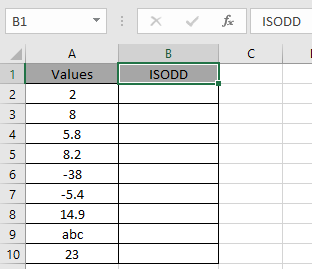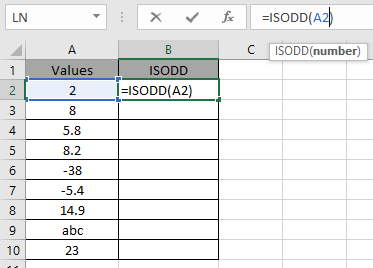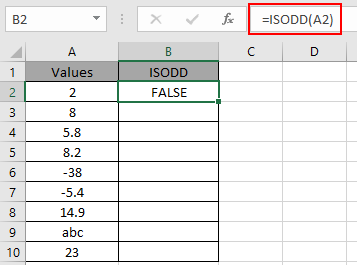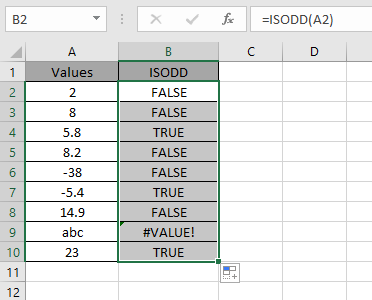# How to use the ISODD Function in Microsoft Excel

ODD numbers are all the numbers which are not divisible by 2 either positive or negative. ODD function in excel returns TRUE if the number is TRUE or FALSE for else number.

ODD function is generally used other functions like ROWS function to traverse through alternate rows. And to highlight alternate rows or elements Conditional formatting with formula uses the ODD function.

ISODD function checks the number in the argument and if the number is ODD it returns TRUE or else it returns FALSE.
Syntax:

 =ISODD ( number )

number : value to the function can be provided as cell reference or directly.
Now let’s understand the function more via using it in an exampleHere we have a list of values and we need to check whether the value is ODD or not.

Use the formula:

 = ISODD ( A2 )

A2 : number provided as cell referenceThe blue box on A2 cell defines that the A2 is cell reference to the ISODD function.2 is not an ODD number so the function returns the logic value TRUE.
Copy the formula to other cells using the Ctrl + D shortcut key.As you can see, the function returns the logic values either TRUE or FALSE. Below are some of the observational results.
Note:

1. All decimal numbers are truncated to integers by the function like - 2.9 truncates to - 2 and 5.7 truncates to 5.
2. The function returns the #VALUE error if the argument to the function is non - numeric value.
3. The function returns the #NAME? error, if the text is given to the input without using quotes.

You can use the ISODD function to highlight the every other using the ROW function.
Use the formula in the conditional formatting with formula option

 = ISEVEN ( ODD ( ) )

Hope you understood how to use ISODD function in Excel 2016. Find more articles on Excel LOGICAL functions like ISODD function here. Please share your query below in the comment box. We will assist you.

Popular Articles

Terms and Conditions of use

The applications/code on this site are distributed as is and without warranties or liability. In no event shall the owner of the copyrights, or the authors of the applications/code be liable for any loss of profit, any problems or any damage resulting from the use or evaluation of the applications/code.